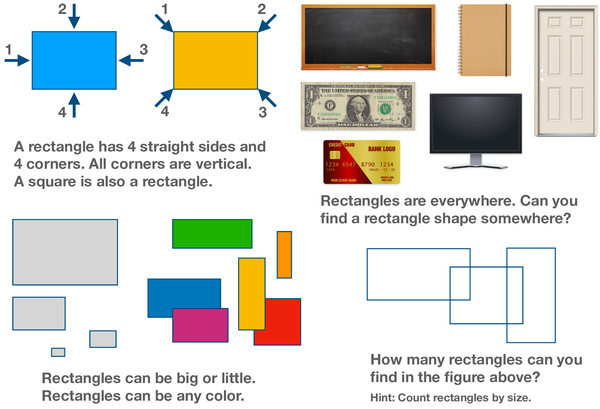# All About Shapes - What is a Rectangle?Shapes are one of the first mathematical subjects introduced to kids. At around 18 months old, kids start to notice differences between shapes. At 2 years old, they begin to understand, recognize and name basic shapes such as circle, triangle, square, and rectangle. Rectangles are one of the most common shapes that form a part of our daily life. A rectangle is 2D shape that has 4 straight sides and 4 corners, and all 4 corners are vertical.

This worksheet is all about rectangles. It helps kids learn about the concepts of rectangles and how to recognize rectangles. A Rectangle is a four sided-polygon, with its parallel sides equal to each other and all four internal angles equal to 90 degrees. An interesting fact to know is that a square is a special type of rectangle, with 4 equal sides. Rectangles can be any size. Rectangles can be any color: yellow, green, red, and so on. Some real-life examples of rectangles are blackboards, notebooks, doors, dollar bills, computer screens, and bank cards. A simple exercise is provided at the end for kids to recognize rectangles. This could help kids reinforce their understanding of the concept of the rectangle shape.